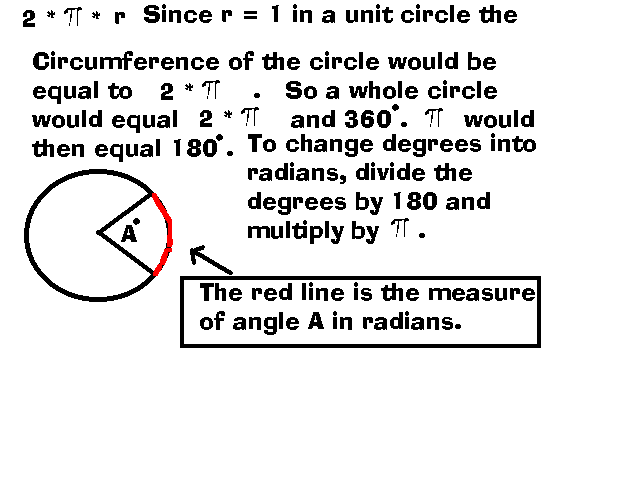+ Text Only Site
+ Non-Flash Version
+ Contact GlennDrag Activity
If so instructed by your teacher, print out a worksheet page for these problems.

Background:

Visit the Glider Trajectory Problem and the Vector Balance of Forces for a Glider. Study the equation:  L/D = 1/a where L stands for lift, approximately equals the weight of the glider, and is measured in newtons; D stands for the drag on the glider and is measured in newtons; and a stands for glide angle and is measured in radians.

Weight and mass are related. Weight is equal to mass times gravity: Fw = m * g where Fw stands for weight and is measured in newtons (Note: W is also used as a symbol for weight.), m stands for mass and is measured in kilograms, and g stands for gravity and is equal to 9.8 m/s2.

Radian measure is another way of measuring the size of an angle. When we measure an angle in radians we are measuring the length of the arc the angle cuts out of a unit circle. A unit circle has a radius of one unit; therefore, the circumference of a unit circle is equal to 2 times pi.Problems:

Create a spreadsheet that finds the weight of each glider, the measure of each angle in degrees, and the drag on each glider at angles of 2, 3, 4, and 5 degrees.

Model Gliders
Mass
Full-sized Gliders
Mass
1
.5 kg
4
500 kg
2
.9 kg
5
900 kg
3
1.4 kg
6
1,400 kg

Questions:

1. Compare the drag between model glider 1 and the full-sized glider 4 for all four angles. What conclusion can you draw?
2. Based on your answer in Question 1, would it be valid to use a model glider to study the drag on a full-sized glider?
3. What is the relationship between glide angle and drag?

Related Pages:
Standards
Worksheet
Lesson Index
Aerodynamics Index+ Inspector General Hotline + Equal Employment Opportunity Data Posted Pursuant to the No Fear Act + Budgets, Strategic Plans and Accountability Reports + Freedom of Information Act + The President's Management Agenda + NASA Privacy Statement, Disclaimer, and Accessibility CertificationEditor: Tom Benson NASA Official: Tom Benson Last Updated: Thu, May 13 02:38:26 PM EDT 2021 + Contact Glenn